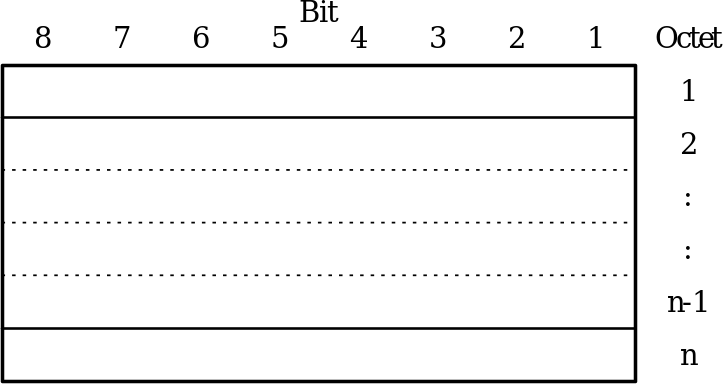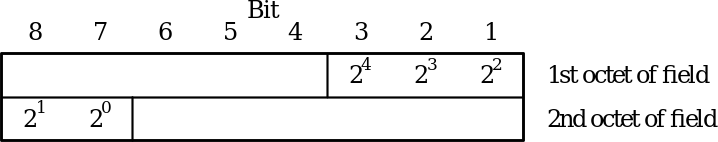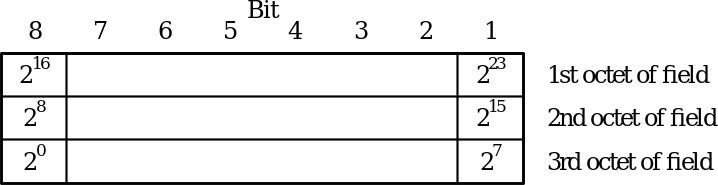## 5 Frame structure

04.643GPPGeneral Packet Radio Service (GPRS)Mobile Station - Serving GPRS Support Node (MS-SGSN) Logical Link Control (LLC) layer specificationTS

## 5.1 General

All logical link control layer peer-to-peer exchanges shall be in frames conforming to the format shown in Figure 3. The frame header shall consist of the address and control fields, and is a minimum of 2 octets and a maximum of 37 octets long.

 8 7 6 5 4 3 2 1 Address Field (1 octet) Control Field (variable length, max. 36 octets) Information Field (variable length, max. N201 octets) Frame Check Sequence Field (3 octets)

Figure 3: LLC frame format

## 5.2 Address field

The address field consists of a single octet. The address field contains the SAPI and identifies the DLCI for which a downlink frame is intended and the DLCI transmitting an uplink frame. The format of the address field is defined in subclause 6.2.

## 5.3 Control field

The control field typically consists of between one and three octets. The SACK supervisory frame also includes a variable-length bitmap field of up to 32 octets. The format of the control field is defined in subclause 6.3.

## 5.4 Information field

The information field of a frame, when present, follows the control field (see subclause 5.4 above). The maximum number of octets in the information field (N201) is defined in subclause 8.9.5.

## 5.5 Frame Check Sequence (FCS) field

The FCS field shall consist of a 24 bit cyclic redundancy check (CRC) code. The CRC-24 is used to detect bit errors in the frame header and information fields.

The FCS field contains the value of a CRC calculation that is performed over the entire contents of the header and information field, except for UI frames transmitted in unprotected mode, in which case the FCS field contains the value of a CRC calculation that is performed over the frame header and the first N202 octets (see subclause 8.9.6) of the information field only (see subclause 6.3.5.5.2). The information over which the CRC is calculated is referred to as the dividend in this subclause. Bit (1, 1) of the dividend is the highest-order term in the calculation (see subclause 5.7.3). CRC calculation shall be done before ciphering at the transmitting side, and after deciphering at the receiving side.

NOTE: The definition below is different from that in 3GPP TS 04.22  only with respect to the variable dividend length k of the LLC frames. In 3GPP TS 04.22, the RLP frame has a fixed dividend length, but the LLC frame has a variable dividend length.

The CRC shall be the ones complement of the sum (modulo 2) of:

– the remainder of xk (x23 + x22 + x21 + + x2 + x + 1) divided (modulo 2) by the generator polynomial, where k is the number of bits of the dividend; and

– the remainder of the division (modulo 2) by the generator polynomial of the product of x24 by the dividend.

The CRC-24 generator polynomial is:

G(x) = x24 + x23 + x21 + x20 + x19 + x17 + x16 + x15 + x13 + x8 + x7 + x5 + x4 + x2 + 1

The result of the CRC calculation is placed within the FCS field as described in subclause 5.7.3.

NOTE: As a typical implementation at the transmitter, the initial content of the register of the device computing the remainder of the division is pre-set to all "1’s" and is then modified by division by the generator polynomial (as described above) of the dividend; the ones complement of the resulting remainder is put into the FCS field.

As a typical implementation at the receiver, the initial content of the register of the device computing the remainder of the division is pre-set to all "1’s". The final remainder, after multiplication by x24 and then division (modulo 2) by the generator polynomial of the received frame, will be (in the absence of errors):

C(x) = x22 + x21 + x19 + x18 + x16 + x15 + x11 + x8 + x5 + x4

## 5.6 Transparency

### 5.6.1 Bit transparency

Because of the frame delimitation technique used in LLC, the frame can include any possible sequence of bits without the need for e.g., bit stuffing as defined in Q.921.

### 5.6.2 Information protection

The information carried within a UI frame may be considered as either "protected" or "unprotected" (see subclause 6.3.5.5.2). CRC error detection procedures are only used on the first octets of the information content within unprotected UI frames, supporting applications that can tolerate bit errors.

### 5.6.3 Octet alignment

LLC provides only an octet-aligned service to layer 3. LLC requires that information exchanged with layer 3 contains an integral number of octets.

## 5.7 Format convention

### 5.7.1 Numbering convention

The basic convention used in the present document is illustrated in Figure 4. The bits are grouped into octets. The bits of an octet are shown horizontally and are numbered from 1 to 8. Multiple octets are shown vertically and are numbered from 1 to n.Figure 4: Format convention

### 5.7.2 Order of transmission

Frames are transferred between the LLC layer and underlying protocol layers in units of octets, in ascending numerical octet order (i.e., octet 1, 2, , n-1, n). The order of bit transmission is specific to the underlying protocols used across the Um interface (e.g., RLC) and the Gb interface (BSSGP).

### 5.7.3 Field mapping convention

When a field is contained within a single octet, the lowest bit number of the field represents the lowest-order value. When a field spans more than one octet, the order of bit values within each octet progressively decreases as the octet number increases. In that part of the field contained in a given octet the lowest bit number represents the lowest-order value.

For example, a bit number can be identified as a couple (o, b) where o is the octet number and b is the relative bit number within the octet. Figure 5 illustrates a field that spans from bit (1, 3) to bit (2, 7). The high-order bit of the field is mapped on bit (1, 3) and the low-order bit is mapped on bit (2, 7).Figure 5: Field mapping convention

An exception to the preceding field mapping convention is the FCS field. In this case bit 1 of the first octet is the high-order bit and bit 8 of the last octet is the low-order bit. The field mapping for a 24 bit FCS is shown in Figure 6Error: Reference source not found.Figure 6: FCS mapping convention

## 5.8 Invalid frames

An invalid frame is a frame that:

– contains fewer octets than necessary to include the address field, control field, information field, and FCS field necessary to constitute a complete frame according to the contents of the control field;

– has the PD bit set to 1;

– contains a reserved SAPI or a SAPI that is not supported or not assigned to a layer‑3 entity; or

– contains an FCS error.

An invalid frame shall be discarded without notification to the sender. No action shall be taken as the result of that frame.